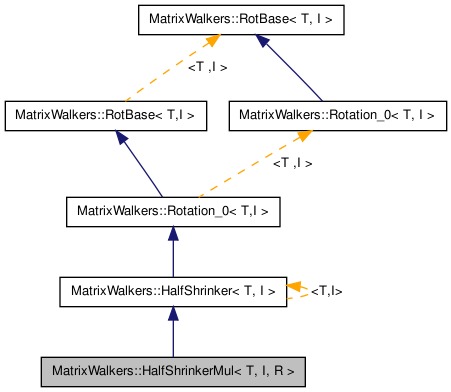MatrixWalkers::HalfShrinkerMul< T, I, R > Struct Template Reference

Similar to HalfShrinker, but instead of multiplying the sums with 0.25 it uses an arbitrary number. More...

Inheritance diagram for MatrixWalkers::HalfShrinkerMul< T, I, R >:[legend]
Collaboration diagram for MatrixWalkers::HalfShrinkerMul< T, I, R >:[legend]

Detailed Description

template<class T, class I = PtrInt, class R = Real> struct MatrixWalkers::HalfShrinkerMul< T, I, R >

Similar to HalfShrinker, but instead of multiplying the sums with 0.25 it uses an arbitrary number.

Definition at line 75 of file saupePredictor.cpp.

The documentation for this struct was generated from the following file:

Generated on Thu Aug 6 22:33:15 2009 for Fractal Image Compressor by1.5.8